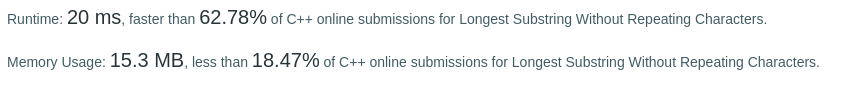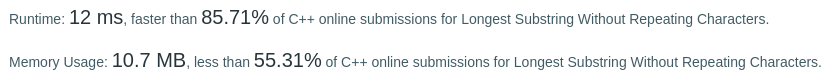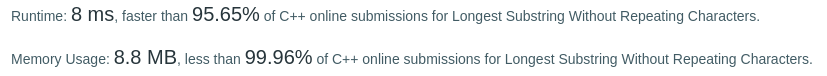# Coding Spirit

0%

Algorithm Puzzles everyday every week sometimes: Longest Substring Without Repeating Characters

## Puzzle

Puzzle from leetcode:

Given a string, find the length of the longest substring without repeating characters.

Example 1:

Input: “abcabcbb”
Output: 3
Explanation: The answer is “abc”, with the length of 3.
Example 2:

Input: “bbbbb”
Output: 1
Explanation: The answer is “b”, with the length of 1.
Example 3:

Input: “pwwkew”
Output: 3
Explanation: The answer is “wke”, with the length of 3.
Note that the answer must be a substring, “pwke” is a subsequence and not a substring.

## Solution

### First came out solution

Traverse substring(not all of them, with a little bit optimization)

Time complexity: O(n^3)But this solution is not good enough since the time complexity is O(n^3). Only better than 62.78% cpp users.

### Sliding Window

Checked the reference answer, there is another solution: using sliding Window.

Time complexity: O(n)It’s better than 85.71% now.

### Assuming ASCII 128

The third way to solve this is assuming the string inputs are all ASCII 128 characters… In this case no hash table is needed.This solution is faster than 95.65%.